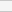GNSS Geodesy Innovation Interviews UAV/UAS Positioning Surveying Applications Perspective Time Navigation Mapping Policy Land Administration News Archives LBS GIS SDI Imaging E-ZineGeodesy

# VLBI geodetic precision with different models

Jun 2008 | Comments Off on VLBI geodetic precision with different models

Erhu WEI, Jingnan LIU, Peijing PAN

## The method of experiment

The data resource
In this experiment, we use the software of OCCAM 5.0 and the data in 2001-2007 computing the corresponding geodetic parameters. Based on the needs of the software, we choose the data of moderate memory (about 1M) to facilitate rapid comparative analysis.

The method of model combination
In order to compare the use of different software models in the results between the levels of accuracy, we classify these models into 6 items:
I In the calculation of the daily and sub-daily change of Earth Orientation Parameters (EOP) from ocean tide term, we choose EANES model; in the calculation of the precession and nutation, we choose IAU1980 nutation model;
II In the calculation of the daily and sub-daily change of Earth Orientation Parameters (EOP) from ocean tide term, we choose EANES model; in the calculation of the precession and nutation, we choose HERRING nutation model;
III In the calculation of the daily and sub-daily change of Earth Orientation Parameters (EOP) from ocean tide term, we choose EANES model; in the calculation of the precession and nutation, we choose MHB_2000 nutation model;
IV In the calculation of the daily and sub-daily change of Earth Orientation Parameters (EOP) from ocean tide term, we choose RAY model; in the calculation of the precession and nutation, we choose IAU1980 nutation model;
V In the calculation of the daily and sub-daily change of Earth Orientation Parameters (EOP) from ocean tide term, we choose RAY model; in the calculation of the precession and nutation, we choose HERRING nutation model;
VI In the calculation of the daily and sub-daily change of Earth Orientation Parameters (EOP) from ocean tide term, we choose RAY model; in the calculation of the precession and nutation, we choose MHB_2000 nutation model;

The criteria of precision compare

In the work of surveying, the power of the observation is the relative numerical indicator which denotes its precision. It is used in weighing various observation of different precision for handling these observations. For one group of observation, if its sigma is smaller, then its power is larger; or if its sigma is larger, its power is smaller. In this paper, the constant “FACTOR” means the value of the limitation for editing the data, the OCCAM software then sets this “FACTOR” value in the control files “KVLBI.OPT” and “KVLBI_ IE.OPT”, it can compute the limitation of the observation delay value subtract the computation delay value. If the difference between observation delay value and computation delay value is bigger than the “FACTOR” multiplies the expectation of the sigma, that is to say the precision obtained from this observation is not good, in this case, we will reduce the power of this observation (explained with * in this paper). If the number of the observations being reduced is too much, then the usability of this observation is small. The data of CHI-SQ is one indicator of reflecting the precision with kalman filter method, if its value is close to 1, then the precision of this result is good.

## The analyze of the experiment result

Based on the combination of the above models, we compute the data following the chronological order:

The compare result by adopting the data of 2001

In calculating the data of the year 2001, after the relevant solution, we choose the better data 010424XE.NGS on April 24 to explain the result. The specific calculating result please refer to table1 (I- VI represent various combination of different models, the figures shown in this table represent the value of CHI-SQ): Table 1 the processing result of 010424XE.NGS

In the data processing on April 24 2001, we can see that if we choose ALGOPARK and FORTLEZA as the reference station for adjustment, in addition to the fourth group, the effects of the others are not ideal. After removing the results of these two stations, we average the other four stations, and then we get the first group of average.

Pages: 1 2 3 4 5(No Ratings Yet)Loading...